# Precalculus : Convert Between Degrees and Radians

## Example Questions

← Previous 1

### Example Question #1 : Convert Between Degrees And Radians

Convert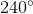to radians.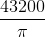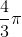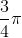Explanation:

In order to solve this problem, we must know that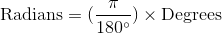with this formula, we can find our answer: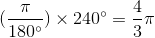### Example Question #1 : Convert Between Degrees And Radians

Convert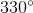to radians.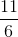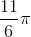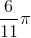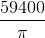Explanation:

In order to solve this problem, we need to know thatusing this formula, we can find our answer: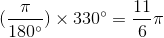### Example Question #2 : Convert Between Degrees And Radians

Convert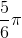to degrees.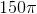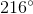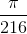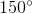Explanation:

In order to solve this problem, we need to know that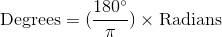using this formula, we can find our answer: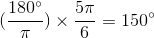### Example Question #3 : Angle Measures In Degrees And Radians

Convertto radians.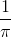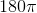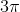Explanation:

To solve this problem, we need to know thatwe can arrive to our answer by using this formula: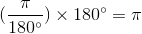### Example Question #71 : Trigonometric Functions

Convert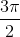to degrees.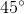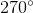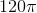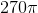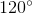Explanation:

In order to solve this problem, we need to know thatwe can arrive to our answer by using this formula: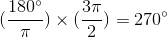### Example Question #7 : Convert Between Degrees And Radians

Findexpressed as radians.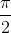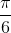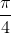Explanation:

To convert from degrees into radians,

multiply the angle in degrees by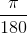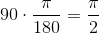### Example Question #8 : Convert Between Degrees And Radians

Convert the following to degrees: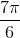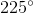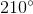Explanation:

To convert, multiply by the conversion factor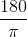.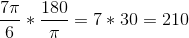### Example Question #9 : Convert Between Degrees And Radians

Convert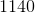degrees into radians.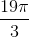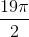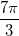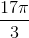Explanation:

To convert between degrees and radians, you must use the following conversion factor 180 degrees = pi radians.

Therefore: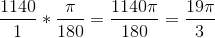### Example Question #10 : Convert Between Degrees And Radians

Please convert the following from degrees to radians: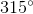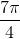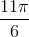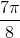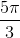Explanation:

To convert from degrees to radians, multiply the input by:In this case: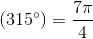### Example Question #11 : Convert Between Degrees And Radians

Please convert the following angle to degrees: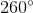Explanation:

To convert from radians to degrees, multiply the input by.

In this case: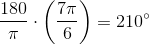← Previous 1

### All Precalculus Resources